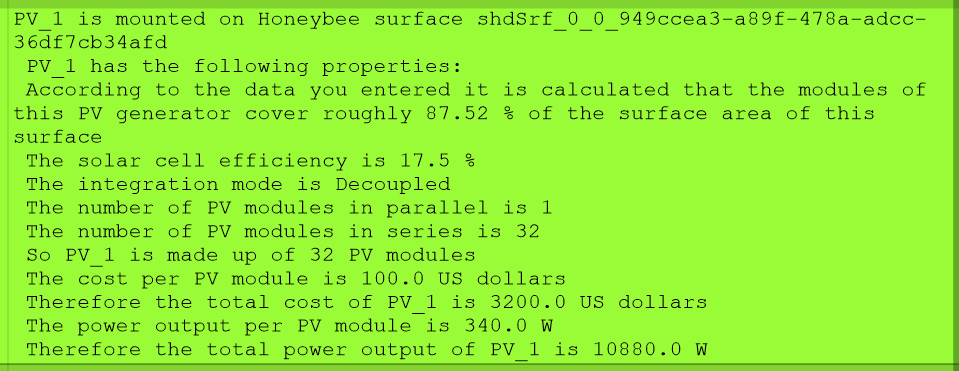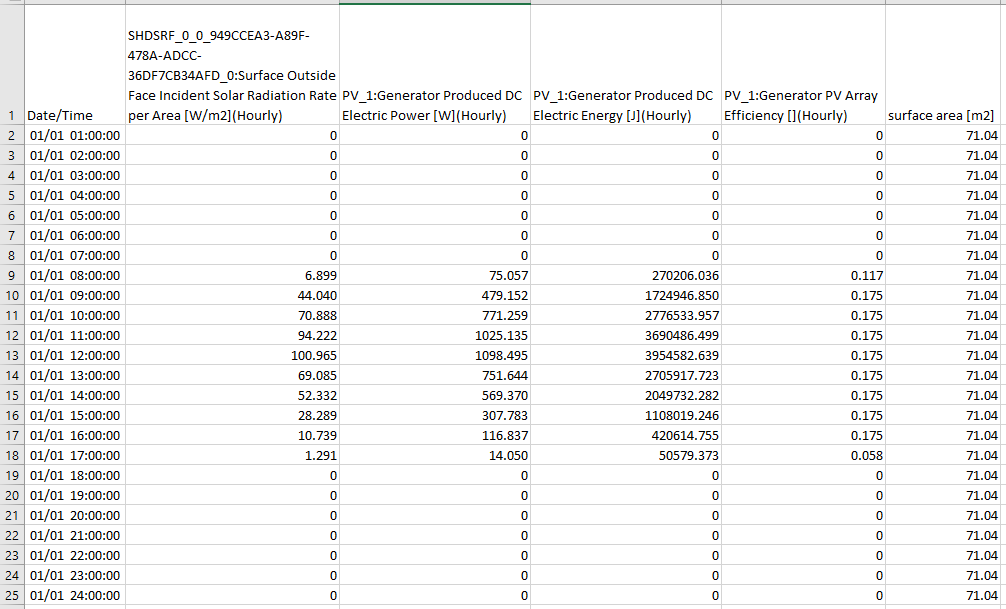Question-and-Answer Resource for the Building Energy Modeling Community
Get started with the Help page

# How is the Generator Produced DC Electric Power calculated?

The following are the output variables for a particular PV surface.

May I ask how is the Generator Produced DC Electric Power [W] calculated?

It seems it is not equal to:

Surface Outside Face Incident Solar Radiation Rate per Area [W/m2]

x Surface Area [m2]

x Generator PV Array Efficiency

The PV generator is specified in Honeybee as the following:The hourly PV output for one day is:edit retag close merge delete

Sort by » oldest newest most voted

You didn't give all parameters so we could actually recreate your numbers, but it's a simple explanation so you'll have no trouble doing it yourself.

You can just read up on this in the Engineering Reference. For a Simple Model, the power output is calculated as  :

$$P = A_{surf} \cdot f_{active} \cdot G_{T} \cdot \eta_{cell} \cdot \eta_{inverter}$$

Where:

more

Thanks, Julien. I realized that the ηcell: the module conversion efficiency or Cell Efficiency is not the Generator PV Array Efficiency as shown in the table above, and I shouldn't use the latter to calculate Generator Produced DC Electric Power.

Now, the numbers add up: e.g. in the case of the hour 8am, the A_surf is 71.04m2, F_active is 87.52%, G_t is 6.899 W/m2, cell efficiency is 17.5% as specified in IDF.

So, the Generator Produced DC Electricity Power is 75.061W = 71.04 * 0.8752 * 6.899 * 0.175, which is quite close to what is shown in the table.

May I ask how Generator PV Array Efficiency is calculated as it is not explained in EnergyPlus document?

I did tell you that already. Generator PV array efficiency is $\eta_{cell}$, it depends on the PhotovoltaicPerformance:XXX object you do use (there are three options: Simple, EquivalentOne-Diode, and Sandia). If you use the PhotovoltaicPerformance:Simple, it depends on these three fields here. In the example they give, they used "Fixed" with Value for Cell Efficiency if Fixed=0.12, so it's always 12%

Because I know that your follow-up question will be "Why is it 0.058 at 17:00 and not 0.175?", that's because you report hourly and not at the timestep level, so it's averaged. If there isn't at least 0.3 W/m2 of irradiance, then the module is off and eff is zero. The CalcSimplePV is dead simple to follow (hence the name), see for yourself at Photovoltaics.cc#L727.

The variable that gets reported by E+ is PVarray( thisPV ).Report.ArrayEfficiency, you'll see it's either Eff (if fixed, that's the value you entered), or if irradiance is below the MinIrradiance, it's zero.